cancel
Showing results for
Did you mean:
See all
Top groups
Explore all groups
Support
It's not the same without you

Join the community to find out what other Atlassian users are discussing, debating and creating.How to calculate total time from creation of Jira incident to closing it?

Can this be done via a Jira query? I need to do this for multiple incidents.

1 accepted

This is not possible natively with Jira automatically.

You'll need to look into an add-on or even some SQL if you have more powerful reporting capability.

ScriptRunner for Jira will be able to achieve what you need in a scripted custom field.

PowerScripts for Jira I am not 100% sure that Power Scripts has similar functionality but it would be worth checking out both options.

I believe there might also be a gadget you could use on a dashboard Resolution Time might be useful for you as well.

Aah OK. Looks like I will need to reach out to our Jira admin to get a custom field created.

Brittany Wispell likes this
import com.atlassian.core.util.DateUtils
DateUtils.getDurationString(((new Date().getTime() - issue.getCreated().time) / 1000) as Long)

This will get you the time since the ticket was created ^^

The below should get the time of how long each ticket was in status.

//Required Imports
import com.atlassian.core.util.DateUtils
import com.atlassian.jira.component.ComponentAccessor
import com.atlassian.jira.issue.history.ChangeItemBean
import com.atlassian.jira.issue.Issue

// Get a pointer to the Change History Manager
def changeHistoryManager = ComponentAccessor.changeHistoryManager

// Ensure that the script field does not store cached values and always dynamically re calculates the values every time a page is laoded.
enableCache = {-> false}

// Define the statuses to report on below
def status1 = "Workflow Status"
def status2 = "Status"
def status3 = "Status"
def status4 = "Status"
def status5 = "Status"
def status6 = "Status"
def status7 = "Status"
def status8 = "Status"

// Get a pointer to the current issue
def issue = issue as Issue

// Get the Suspect time
List<Long> rt1 = [0L]
changeHistoryManager.getChangeItemsForField (issue, "status").reverse().each {ChangeItemBean item ->

def timeDiff = System.currentTimeMillis() - item.created.getTime()
if (item.fromString == status1) {
rt1 << -timeDiff
}
if (item.toString == status1){
rt1 << timeDiff
}

}

// Get the Step 2 Time
List<Long> rt2 = [0L]
changeHistoryManager.getChangeItemsForField (issue, "status").reverse().each {ChangeItemBean item ->

def timeDiff = System.currentTimeMillis() - item.created.getTime()
if (item.fromString == status2) {
rt2 << -timeDiff
}
if (item.toString == status2){
rt2 << timeDiff
}

}

// Get the Step 3 Time
List<Long> rt3 = [0L]
changeHistoryManager.getChangeItemsForField (issue, "status").reverse().each {ChangeItemBean item ->

def timeDiff = System.currentTimeMillis() - item.created.getTime()
if (item.fromString == status3) {
rt3 << -timeDiff
}
if (item.toString == status3){
rt3 << timeDiff
}

}

// Get the Step 4 Time
List<Long> rt4 = [0L]
changeHistoryManager.getChangeItemsForField (issue, "status").reverse().each {ChangeItemBean item ->

def timeDiff = System.currentTimeMillis() - item.created.getTime()
if (item.fromString == status4) {
rt4 << -timeDiff
}
if (item.toString == status4){
rt4 << timeDiff
}

}

// Get the Step 5 Time
List<Long> rt5 = [0L]
changeHistoryManager.getChangeItemsForField (issue, "status").reverse().each {ChangeItemBean item ->

def timeDiff = System.currentTimeMillis() - item.created.getTime()
if (item.fromString == status5) {
rt5 << -timeDiff
}
if (item.toString == status5){
rt5 << timeDiff
}

}

// Get the Step 6 Time
List<Long> rt6 = [0L]
changeHistoryManager.getChangeItemsForField (issue, "status").reverse().each {ChangeItemBean item ->

def timeDiff = System.currentTimeMillis() - item.created.getTime()
if (item.fromString == status6) {
rt6 << -timeDiff
}
if (item.toString == status6){
rt6 << timeDiff
}

}

// Get the Step 7 Time
List<Long> rt7 = [0L]
changeHistoryManager.getChangeItemsForField (issue, "status").reverse().each {ChangeItemBean item ->

def timeDiff = System.currentTimeMillis() - item.created.getTime()
if (item.fromString == status7) {
rt7 << -timeDiff
}
if (item.toString == status7){
rt7 << timeDiff
}

}

// Get the Step 8 Time
List<Long> rt8 = [0L]
changeHistoryManager.getChangeItemsForField (issue, "status").reverse().each {ChangeItemBean item ->

def timeDiff = System.currentTimeMillis() - item.created.getTime()
if (item.fromString == status8) {
rt8 << -timeDiff
}
if (item.toString == status8){
rt8 << timeDiff
}

}

// Return times for each status
// NOTE: doesn't show anything if less than 60 seconds
def status1Time = DateUtils.getDurationString(Math.round((rt1.sum().toString().toDouble() / 1000) as Double)) ?: "0m" as String
def status2Time = DateUtils.getDurationString(Math.round((rt2.sum().toString().toDouble() / 1000) as Double)) ?: "0m" as String
def status3Time = DateUtils.getDurationString(Math.round((rt3.sum().toString().toDouble() / 1000) as Double)) ?: "0m" as String
def status4Time = DateUtils.getDurationString(Math.round((rt4.sum().toString().toDouble() / 1000) as Double)) ?: "0m" as String
def status5Time = DateUtils.getDurationString(Math.round((rt5.sum().toString().toDouble() / 1000) as Double)) ?: "0m" as String
def status6Time = DateUtils.getDurationString(Math.round((rt6.sum().toString().toDouble() / 1000) as Double)) ?: "0m" as String
def status7Time = DateUtils.getDurationString(Math.round((rt7.sum().toString().toDouble() / 1000) as Double)) ?: "0m" as String
def status8Time = DateUtils.getDurationString(Math.round((rt8.sum().toString().toDouble() / 1000) as Double)) ?: "0m" as String

return " Workflow Status = " + status1Time + "<br/>"+ " Workflow Status = " + status2Time + "<br/>"+ " Workflow Status = " + status3Time + "<br/>"+ " Workflow Status = " + status4Time + "<br/>"+ " Workflow Status = " + status5Time + "<br/>"+ " Workflow Status = " + status6Time

# people like this

This would benefit me greatly, but I'm new to Jira (SD) so... how do you integrate the above into Jira SD? i.e. do you need to add that into a custom field etc etc

Explain it like I'm 5 :P

Check to see if you have ScriptRunner for Jira in your add-ons sections.

How to add a scripted field after confirming you have Adaptavist ScriptRunner for Jira

Settings > Add-ons > Script Fields > Custom Script Field

Below are images of the steps to get to the scripted custom field section.

1.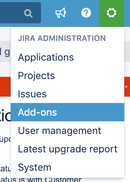2.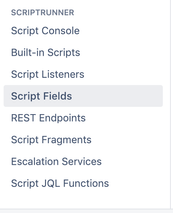3.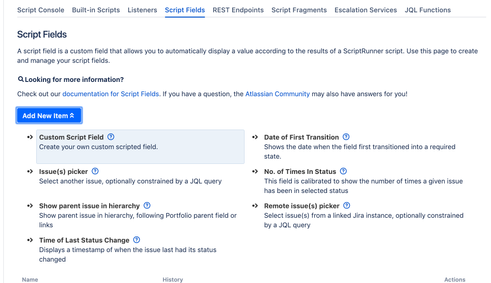4.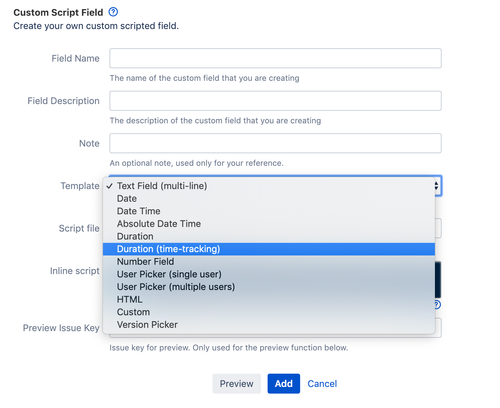5.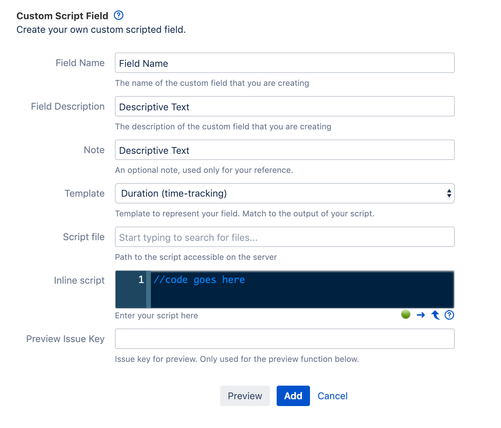Let me know if you have any other questions or need more clarification on how to set this up! I'd be happy to help.

Hi.  Step 5 shows as blank - should it? I've followed the steps and it doesn't appear to be working as expected.

I've named the field "status duration" and the field gets populated with:

I'm very new to Jira and Jira SD, so I apologize if everyone reading this is shaking their heads :P

Ah! Okay.

So how many statues do you have that you are trying to get the duration for?

Would you mind sending me your statues and I can update the code per your use case?

Step 5 is this one.It's just formatted weird.

It would be:

Waiting for Support

Waiting for Customer

Pending

In Progress

Would I be right in saying that I should change the "status" in the script so that it reads

def status2 = "Waiting for Support"
def status3 = "Waiting for Customer"
def status4 = "Pending"
def status5 = "In Progress"

and then change each reference to "status" (in green) to the relevant actual status as above?

Yes anywhere that has "Status" or "Workflow Status" you will need to change to the statues per your workflow.

Ok - so does the initial "workflow status" need to change as well? And does the case of the statuses matter? i.e. is Waiting for Customer handled differently to waiting for customer?

Yes. def status1 would be your initial workflow status. :)

I do not think case would be an issue BUT if one way doesn't work try the other.

Hmm... I still get the issue that I had initially which makes me think I'm doing something wrong at a more basic level.

Basically I just need to track four status types:

Waiting for Support

Waiting for Customer

Pending

In Progress

Could you send me screenshots of the custom field configuration?

When the ticket is initially opened what is the status?

Try the below. I have added all your statues to this script below.

//Required Imports
import com.atlassian.core.util.DateUtils
import com.atlassian.jira.component.ComponentAccessor
import com.atlassian.jira.issue.history.ChangeItemBean
import com.atlassian.jira.issue.Issue

// Get a pointer to the Change History Manager
def changeHistoryManager = ComponentAccessor.changeHistoryManager

// Ensure that the script field does not store cached values and always dynamically re calculates the values every time a page is laoded.
enableCache = {-> false}

// Define the statuses to report on below
def status1 = "Waiting for Support"
def status2 = "Waiting for Customer"
def status3 = "Pending"
def status4 = "In Progress"

// Get a pointer to the current issue
def issue = issue as Issue

// Get the Suspect time
List<Long> rt1 = [0L]
changeHistoryManager.getChangeItemsForField (issue, "status").reverse().each {ChangeItemBean item ->

def timeDiff = System.currentTimeMillis() - item.created.getTime()
if (item.fromString == status1) {
rt1 << -timeDiff
}
if (item.toString == status1){
rt1 << timeDiff
}

}

// Get the Step 2 Time
List<Long> rt2 = [0L]
changeHistoryManager.getChangeItemsForField (issue, "status").reverse().each {ChangeItemBean item ->

def timeDiff = System.currentTimeMillis() - item.created.getTime()
if (item.fromString == status2) {
rt2 << -timeDiff
}
if (item.toString == status2){
rt2 << timeDiff
}

}

// Get the Step 3 Time
List<Long> rt3 = [0L]
changeHistoryManager.getChangeItemsForField (issue, "status").reverse().each {ChangeItemBean item ->

def timeDiff = System.currentTimeMillis() - item.created.getTime()
if (item.fromString == status3) {
rt3 << -timeDiff
}
if (item.toString == status3){
rt3 << timeDiff
}

}

// Get the Step 4 Time
List<Long> rt4 = [0L]
changeHistoryManager.getChangeItemsForField (issue, "status").reverse().each {ChangeItemBean item ->

def timeDiff = System.currentTimeMillis() - item.created.getTime()
if (item.fromString == status4) {
rt4 << -timeDiff
}
if (item.toString == status4){
rt4 << timeDiff
}

}

// Return times for each status
// NOTE: doesn't show anything if less than 60 seconds
def status1Time = DateUtils.getDurationString(Math.round((rt1.sum().toString().toDouble() / 1000) as Double)) ?: "0m" as String
def status2Time = DateUtils.getDurationString(Math.round((rt2.sum().toString().toDouble() / 1000) as Double)) ?: "0m" as String
def status3Time = DateUtils.getDurationString(Math.round((rt3.sum().toString().toDouble() / 1000) as Double)) ?: "0m" as String
def status4Time = DateUtils.getDurationString(Math.round((rt4.sum().toString().toDouble() / 1000) as Double)) ?: "0m" as String

return " Waiting for Support = " + status1Time + "<br/>"+ " Waiting for Customer = " + status2Time + "<br/>"+ " Pending = " + status3Time + "<br/>"+ " In Progress = " + status4Time

Sorry I misunderstood your question earlier. You don't need to fill out the "green" status with the status name. it needed to stay as status.

the 'return' needed to have the status names, same with when you are defining the statuses in def status1 etc.

Thanks - I've entered the revised code. When using a ticket to review the outcome I still get:

(i.e. this hasn't changed)

Hi.   The "Template" in Figure 4 is "Duration (Time Tracking)," but it *should* be "Text Field (multi-line)."

I'm now getting results, have yet to check whether they're what I expect.

Ok... Progress (of sorts).   I've created a new request and moved it through the different statuses.

Although "In Progress" and "Pending" work perfectly, neither "Waiting for Support" nor "Waiting for Customer" are updating - they show 0m regardless of how long/how many times the requests have been in that status....

Okay - it now *almost* works! All but "Waiting for support" now show the correct total times. I *did* have an issue where neither was working, but I changed the case to match that given in the workflow diagram boxes, and that worked for "Waiting for customer" but  it didn't work for "Waiting for support."

So 3/4 of the way there, but not there yet :P

Brittany Wispell likes this

Is waiting for support the initial status?

Yes - customer raises a request and it then goes into "waiting for support."

A request can go backwards and forwards from this status throughout the request's life.

I tried the above-mentioned method below is my edited code

//Required Imports
import com.atlassian.core.util.DateUtils
import com.atlassian.jira.component.ComponentAccessor
import com.atlassian.jira.issue.history.ChangeItemBean
import com.atlassian.jira.issue.Issue
import org.joda.time.Days
import org.joda.time.LocalDate

// Get a pointer to the Change History Manager
def changeHistoryManager = ComponentAccessor.changeHistoryManager

// Ensure that the script field does not store cached values and always dynamically re calculates the values every time a page is laoded.
enableCache = {-> false}

// Define the statuses to report on below
def status1 = "SIT REVIEW"
def status2 = "UAT IN PROGRESS"
def status3 = "UAT END USER"
def status4 = "DEPLOYED-PROD"

// Get a pointer to the current issue
def issue = issue as Issue

// Get the Suspect time
List<Long> rt1 = [0L]
changeHistoryManager.getChangeItemsForField (issue, "status").reverse().each {ChangeItemBean item ->

def timeDiff = System.currentTimeMillis() - item.created.getTime()
if (item.fromString == status1) {
rt1 << -timeDiff
}
if (item.toString == status1){
rt1 << timeDiff
}

}

// Get the Step 2 Time
List<Long> rt2 = [0L]
changeHistoryManager.getChangeItemsForField (issue, "status").reverse().each {ChangeItemBean item ->

def timeDiff = System.currentTimeMillis() - item.created.getTime()
if (item.fromString == status2) {
rt2 << -timeDiff
}
if (item.toString == status2){
rt2 << timeDiff
}

}

// Get the Step 3 Time
List<Long> rt3 = [0L]
changeHistoryManager.getChangeItemsForField (issue, "status").reverse().each {ChangeItemBean item ->

def timeDiff = System.currentTimeMillis() - item.created.getTime()
if (item.fromString == status3) {
rt3 << -timeDiff
}
if (item.toString == status3){
rt3 << timeDiff
}

}

// Get the Step 4 Time
List<Long> rt4 = [0L]
changeHistoryManager.getChangeItemsForField (issue, "status").reverse().each {ChangeItemBean item ->

def timeDiff = System.currentTimeMillis() - item.created.getTime()
if (item.fromString == status4) {
rt4 << -timeDiff
}
if (item.toString == status4){
rt4 << timeDiff
}

}
/*
// Get the Step 5 Time
List<Long> rt5 = [0L]
changeHistoryManager.getChangeItemsForField (issue, "status").reverse().each {ChangeItemBean item ->

def timeDiff = System.currentTimeMillis() - item.created.getTime()
if (item.fromString == status5) {
rt5 << -timeDiff
}
if (item.toString == status5){
rt5 << timeDiff
}

}

// Get the Step 6 Time
List<Long> rt6 = [0L]
changeHistoryManager.getChangeItemsForField (issue, "status").reverse().each {ChangeItemBean item ->

def timeDiff = System.currentTimeMillis() - item.created.getTime()
if (item.fromString == status6) {
rt6 << -timeDiff
}
if (item.toString == status6){
rt6 << timeDiff
}

}

// Get the Step 7 Time
List<Long> rt7 = [0L]
changeHistoryManager.getChangeItemsForField (issue, "status").reverse().each {ChangeItemBean item ->

def timeDiff = System.currentTimeMillis() - item.created.getTime()
if (item.fromString == status7) {
rt7 << -timeDiff
}
if (item.toString == status7){
rt7 << timeDiff
}

}

// Get the Step 8 Time
List<Long> rt8 = [0L]
changeHistoryManager.getChangeItemsForField (issue, "status").reverse().each {ChangeItemBean item ->

def timeDiff = System.currentTimeMillis() - item.created.getTime()
if (item.fromString == status8) {
rt8 << -timeDiff
}
if (item.toString == status8){
rt8 << timeDiff
}

}
*/

// Return times for each status
// NOTE: doesn't show anything if less than 60 seconds
def status1Time = DateUtils.getDurationString(Math.round((rt1.sum().toString().toDouble() / 1000) as Double)) ?: "0m" as String
def status2Time = DateUtils.getDurationString(Math.round((rt2.sum().toString().toDouble() / 1000) as Double)) ?: "0m" as String
def status3Time = DateUtils.getDurationString(Math.round((rt3.sum().toString().toDouble() / 1000) as Double)) ?: "0m" as String
def status4Time = DateUtils.getDurationString(Math.round((rt4.sum().toString().toDouble() / 1000) as Double)) ?: "0m" as String
//def status5Time = DateUtils.getDurationString(Math.round((rt5.sum().toString().toDouble() / 1000) as Double)) ?: "0m" as String
//def status6Time = DateUtils.getDurationString(Math.round((rt6.sum().toString().toDouble() / 1000) as Double)) ?: "0m" as String
//def status7Time = DateUtils.getDurationString(Math.round((rt7.sum().toString().toDouble() / 1000) as Double)) ?: "0m" as String
//def status8Time = DateUtils.getDurationString(Math.round((rt8.sum().toString().toDouble() / 1000) as Double)) ?: "0m" as String

return " SIT REVIEW = " + status1Time + "<br/>"+ " UAT IN PROGRESS = " + status2Time + "<br/>"+ " UAT END USER = " + status3Time + "<br/>"+ " DEPLOYED-PROD = " + status4Time

When  I try with the Template "Duration (time-tracking)" I'm getting an error

When I try with Text Field (Multi-line) I'm getting the below-shown output

SIT REVIEW = 0m
UAT IN PROGRESS = 0m
UAT END USER = 0m
DEPLOYED-PROD = 0m

My end goal is to get the time spent in days on each workflow status

thanks

I was pretty happy to find this, unfortunately, I wasn't able to get this working in a reasonable amount of time either.  Looking for other options now.

I have a tool I developed using Google Sheets that does what you need. It will pull the data from Jira and automatically calculate time in status along with quite a few other metrics.  Here is my blog post explaining the tool.